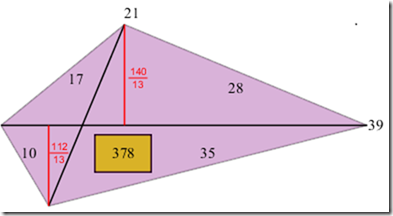Problems & Puzzles: Problems

Dmitry Kamenetsky sent the following nice puzzle:

Can you find a convex quadrilateral such that all four of its sides and two diagonals have lengths such that:

Q1. The quadrilateral has the smallest area and all four of its sides, two diagonals and area are distinct integers?

BTW, Dimitry has already sent one solutions to Q1 that I will show next week.

Q2. The quadrilateral has the smallest area and all four of its sides, two diagonals and area are distinct prime integers?

Contributions came from Jan van Delden, Emmanuel Vantieghem and Dmitry Kamenetski. BTW, the three of them found the same minimal area solution for Q1 (see diagram below).

***

Jan wrote:

Q1:Q2:

The quadrilateral with diagonals having integer lengths is composed of 4 Heronian triangles (top/down and left/right).

An Herionian triangle is a triangle where the lengths of the sides are integer.
It is known that at least one side has an even length and the lengths 1 and 2 are not possible.
Therefore there is no Heronian triangle with all sides prime. Furthermore the area is always divisible by 6, so a prime area is not feasible

***

Emmanuel wrote:

Q1. My 'champion' is the quadrilateral with sides  10, 17, 28, 35,  diagonals  21, 39  and area  378.

(the diagonal with length  21  is the one that meets the sides  10, 35).

Q2. This is impossible.
In my opinion, the simplest way to prove this is the following :
Suppose  ABCD  is a quadrilateral with sides  a = AB, b = BC, c = CD, d = DA  and diagonals  p = AC, q = BD.
Suppose all these quantities are prime.
It is clear that the prime 2 is not involved here (an integeral triangle that has one side equal to 2 has at least one other side even).
Let  s = (a+b+c+d)/2  be the semiperimeter of  ABCD.  The area  k  of  ABCD  then can be computed by the formula
k = SQRT( (s - a) (s - b) (s - c) (s - d) - (a c + b d + p q) (a c + b d - p q)/4 ).
It is immediately clear that the expression under the root cannot be an integer when  a,b,c,d,p, q  are odd. Thus, "all quantities q, b, c, d, p, q odd" is impossible.

***

Dmitry

For Q2, I have searched all quadrilaterals with sides less than 1500. I have only managed to find two solutions that give a concave (not convex) quadrilateral with prime edges. Here is one with the smallest NON INTEGER area:

A=(0.0,0.0), B=(-1051.0,0.0), C=(-625.0965746907707,1231.4281433806302), D=(-590.8092293054234,50.925971444162)

then AB=1051, BC=1303, CD=1181, AD=593, AC=1381 and BD=463, area=299262.75833496224 (*)

In other news, a guy on the Russian dxdy forum has proved that a solution for problem 2 is impossible. He proved that if a quadrilateral has prime sides and diagonals then it cannot have a prime area. It can perhaps, have an integer non-prime area.... Hopefully he will send you a translation. If not then I will translate it for you later.

BTW, Dmitry not only found the same minimal solution for Q1 than Jan and Emmanuel but he also sent a integer solution for a pentagon! that will be posted in another separate problem.

Note (*) Emmanuel Vantieghem has shown that this solution is impossible for any quadrilateral. See his comments to Q2 in Problem 65.

***Records   |  Conjectures  |  Problems  |  PuzzlesHome | Melancholia | Problems & Puzzles | References | News | Personal Page | Puzzlers | Search | Bulletin Board | Chat | Random Link Copyright © 1999-2012 primepuzzles.net. All rights reserved.